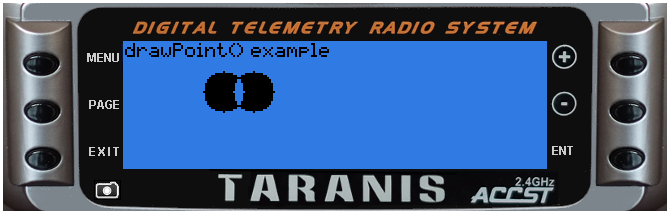## lcd.drawPoint(x, y)

Draws a single pixel at (x,y) position

@status current Introduced in 2.0.0

#### Parameters

• `x` (positive number) x position

• `y` (positive number) y position

#### Return value

none

##### Notice

Taranis has an LCD display width of 212 pixels and height of 64 pixels. Position (0,0) is at top left. Y axis is negative, top line is 0, bottom line is 63. Drawing on an existing black pixel produces white pixel (TODO check this!)

### Examples

lcd/drawPoint-example

``````local function circle(xCenter, yCenter, radius)
local y, x
for y=-radius, radius do
for x=-radius, radius do
if(x*x+y*y <= radius*radius) then
lcd.drawPoint(xCenter+x, yCenter+y)
end
end
end
end

local function run(event)
lcd.clear()
lcd.drawText(1,1,"drawPoint() example", 0)
circle(50, 25, 10)
circle(65, 25, 10)
end

return{run=run}
``````